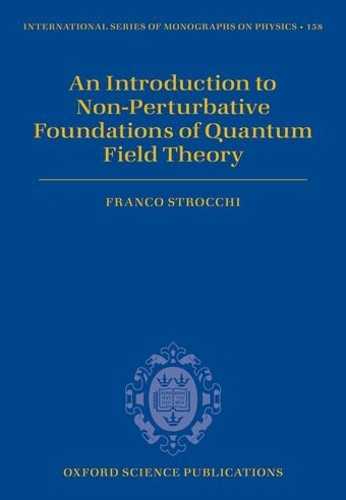•# An Introduction to Non-Perturbative Foundations of Quantum Field Theory - International Series of Monographs on Physics 158 (Hardback)

(author)
£83.00
Hardback 272 Pages / Published: 14/02/2013
• We can order this

Usually dispatched within 1 week

Quantum Field Theory (QFT) has proved to be the most useful strategy for the description of elementary particle interactions and as such is regarded as a fundamental part of modern theoretical physics. In most presentations, the emphasis is on the effectiveness of the theory in producing experimentally testable predictions, which at present essentially means Perturbative QFT. However, after more than fifty years of QFT, we still are in the embarrassing situation of not knowing a single non-trivial (even non-realistic) model of QFT in 3+1 dimensions, allowing a non-perturbative control. As a reaction to these consistency problems one may take the position that they are related to our ignorance of the physics of small distances and that QFT is only an effective theory, so that radically new ideas are needed for a consistent quantum theory of relativistic interactions (in 3+1 dimensions). The book starts by discussing the conflict between locality or hyperbolicity and positivity of the energy for relativistic wave equations, which marks the origin of quantum field theory, and the mathematical problems of the perturbative expansion (canonical quantization, interaction picture, non-Fock representation, asymptotic convergence of the series etc.). The general physical principles of positivity of the energy, Poincare' covariance and locality provide a substitute for canonical quantization, qualify the non-perturbative foundation and lead to very relevant results, like the Spin-statistics theorem, TCP symmetry, a substitute for canonical quantization, non-canonical behaviour, the euclidean formulation at the basis of the functional integral approach, the non-perturbative definition of the S-matrix (LSZ, Haag-Ruelle-Buchholz theory). A characteristic feature of gauge field theories is Gauss' law constraint. It is responsible for the conflict between locality of the charged fields and positivity, it yields the superselection of the (unbroken) gauge charges, provides a non-perturbative explanation of the Higgs mechanism in the local gauges, implies the infraparticle structure of the charged particles in QED and the breaking of the Lorentz group in the charged sectors. A non-perturbative proof of the Higgs mechanism is discussed in the Coulomb gauge: the vector bosons corresponding to the broken generators are massive and their two point function dominates the Goldstone spectrum, thus excluding the occurrence of massless Goldstone bosons. The solution of the U(1) problem in QCD, the theta vacuum structure and the inevitable breaking of the chiral symmetry in each theta sector are derived solely from the topology of the gauge group, without relying on the semiclassical instanton approximation.

Publisher: Oxford University Press
ISBN: 9780199671571
Number of pages: 272
Weight: 694 g
Dimensions: 247 x 178 x 19 mm

MEDIA REVIEWS
This is the book many mathematically inclined theoretical physicists and physics-oriented mathematicians have been waiting for; an introductory textbook on quantum field theory and its applications written by theoretical physicist F. Strocchi, a world expert on the mathematical foundations of relativistic quantum field theory, working in this field himself for decades and having made profound contributions of research results. His book is the answer to the question students or researchers often ask: "Which book would you recommend to learn about quantum field theory?" * Volker Bach, Technische Universitat Braunschweig *
Strocchi's book is an immensely valuable addition to the introductory literature on quantum field theory. It will be useful to physicists, mathematicians, and philosophers of physics alike, as it successfully bridges the gap between the rigorous and the non-rigorous, the formal and the heuristic approaches to quantum field theory. Those looking for a mathematically well-founded approach to the theories combined in the Standard Model of elementary particle physics can hardly do better than consult Strocchi's work. * Simon Friederich, Universitat Goettingen *
This excellent new book presents the fundamental concepts of quantum field theory at a more mathematical and rigorous level than found in the many standard graduate texts in the field. It will be a valuable reference for particle physicists and others seeking a deeper understanding of the theoretical foundations of the standard model. * Graham Shore, Swansea University *